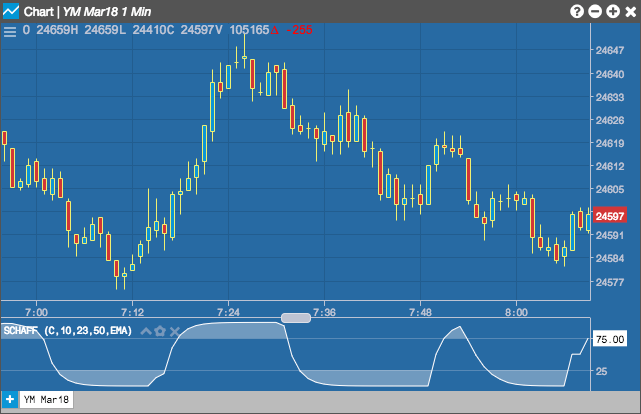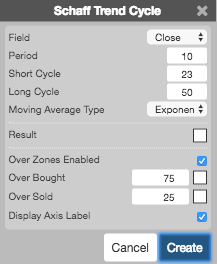Charts

# Schaff Trend Cycle (STC)

The Schaff Trend Cycle (STC) indicator is an oscillator that provides buy and sell signals for trading and identifies market trends. Buy and sell points are typically set at 25 levels upward (buy) and 75 levels downward (sell).## Configuration Options• Field: Price or combination of prices to use as the base for average calculations. Possible values include:
• Open
• High
• Low
• Close
• HL/2 $$\left ( \frac{High + Low}{2} \right )$$
• HLC/3 $$\left ( \frac{High + Low + Close}{3} \right )$$
• HLCC/4 $$\left ( \frac{High + Low + Close + Close}{4} \right )$$
• OHLC/4 $$\left ( \frac{Open + High + Low + Close}{4} \right )$$
• Period: Number of bars to use in the calculations.
• Short Cycle: Length of the long-moving average period.
• Long Cycle: Length for the short-moving average period.
• Moving Average Type: Type of moving average to use in the calculations:
• Simple
• Exponential
• Time Series
• Triangular
• Variable
• VIDYA
• Weighted
• Welles Winder
• Hull
• Double Exponential
• Triple Exponential
• Color Selectors: Colors to use for graph elements.
• Over Zones Enabled: Whether to shade the area between the plot and the horizontal overbought and oversold levels.
• Over Bought: Overbought quantity
• Over Sold: Oversold quantity
• Display Axis Label: Whether to display the most recent value on the Y axis.